Latest Banking jobs   »   Quantitative Aptitude Quiz For SBI/IBPS PO...

# Quantitative Aptitude Quiz For SBI/IBPS PO Prelims 2021- 8th November

Directions (1-5): The following questions are accompanied by two statements (I) and (II). You have to determine which statements(s) is/are sufficient/necessary to answer the questions.
(a) Statement I alone is sufficient to answer the question but statement II alone is not sufficient to answer the question.
(b) Statement II alone is sufficient to answer the question but statement I alone is not sufficient to answer the question.
(c) Both the statements taken together are necessary to answer the question, but neither of the statements alone is sufficient to answer the question.
(d) Either statement I or statement II by itself is sufficient to answer the question.
(e) Statements I and II taken together are not sufficient to answer the question.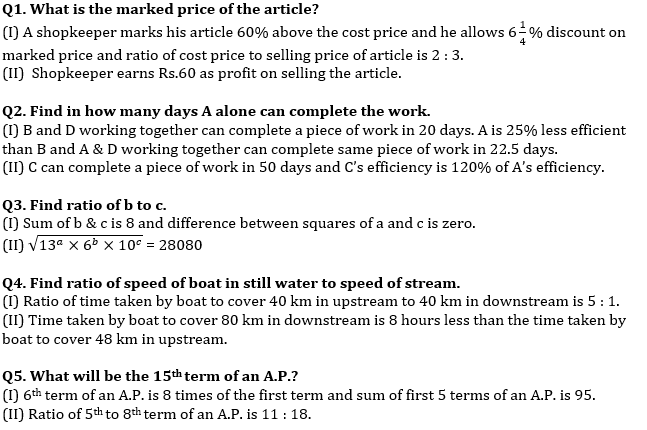Directions (6 -10): The following questions are accompanied by two statements (I) and (II). You have to determine which statements(s) is/are sufficient/necessary to answer the questions.
(a) Statement (I) alone is sufficient to answer the question but statement (II) alone is not sufficient to answer the questions.
(b) Statement (II) alone is sufficient to answer the question but statement (I) alone is not sufficient to answer the question.
(c) Both the statements taken together are necessary to answer the questions, but neither of the statements alone is sufficient to answer the question.
(d) Either statement (I) or statement (II) by itself is sufficient to answer the question.
(e) Statements (I) and (II) taken together are not sufficient to answer the question.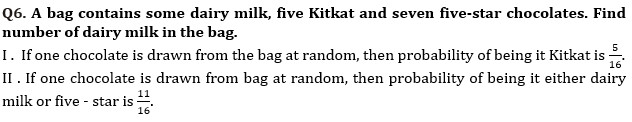Q7. A shopkeeper bought a table at the price of Rs ‘2X’. Find the profit of shopkeeper on selling table (In Rs.)
I . Shopkeeper sold table at 20% profit after allowing two successive discounts of 20% & 4% respectively and difference between marked price and cost price is Rs. 1620.
II . Cost price of a chair is 75% of cost price of that table and difference between their cost price is Rs. 720. Shopkeeper marks up table by 56.25% and allows a discount of 23.2% on it.

Q8. How many men and women are working on this task?
I. (x – 4) men work for first (x – 8) days, after that (x+2) women complete the remaining work in (x – 8) days.
II. Ratio between work did by men to work did by women is 2 : 3 and efficiency of a man and a woman is equal.

Q9. If smaller train added three more compartments of 15 m each, then find in what time both trains cross each other running in opposite direction
I . Length of longer train is 25% more than that of smaller train and speed of longer train & smaller train is 81 km/hr & 108 km/hr respectively.
II . Longer & smaller train cross each other in 36 sec running in the same direction.

Q10. In a river there are two steamers A and B, where A covers 30 km downstream and B covers 30 km upstream. find the speed of B in still water.
I. B takes 2 hours more than A in covering the given distance and their respective directions and sum of still water speed of both the steamers is 16 km/hr.
II. Speed of current is 1 km/hr.

Directions (11-15): In the given questions, two quantities are given, one as ‘Quantity I’ and another as ‘Quantity II’. You must determine relationship between two quantities and choose the appropriate option: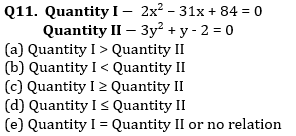Q12. Quantity I – On selling 21 bottles at Rs. 1620, there is a profit equal to the cost price of 6 bottles. The cost price of a bottle is
Quantity II – A, B and C completed a work costing Rs. 2400. A, B and C can complete the work alone in 12, 20 and 30 days respectively. If A, B and C together finish the work, then find the half of per day wage of B?
(a) Quantity I < Quantity II
(b) Quantity I > Quantity II
(c) Quantity I ≥ Quantity II
(d) Quantity I ≤ Quantity II
(e) Quantity I = Quantity II or no relation

Q13. Quantity I – 6499 + 3601 × 14.989 – 8799.9+97.334 =?, approximate value of question mark (?).
Quantity II – Ram invested Rs. 30000 at the rate of X% for two years on compound interest and gets total amount of Rs. 39675. If Ram invested same amount at the rate of (X + 5)% for two years on compound interest, then find four times of interest that Ram will get?
(a) Quantity I > Quantity II
(b) Quantity I < Quantity II
(c) Quantity I ≥ Quantity II
(d) Quantity I ≤ Quantity II
(e) Quantity I = Quantity II or no relation

Q14. A train running at the speed of 108 km/hr crosses a pole in 8 sec.
Quantity I – Length of train.
Quantity II – Length of platform which train crosses in 15 sec.
(a) Quantity I > Quantity II
(b) Quantity I < Quantity II
(c) Quantity I ≥ Quantity II
(d) Quantity I ≤ Quantity II
(e) Quantity I = Quantity II or no relation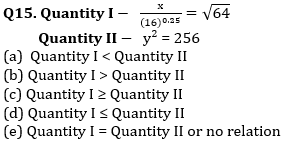Solutions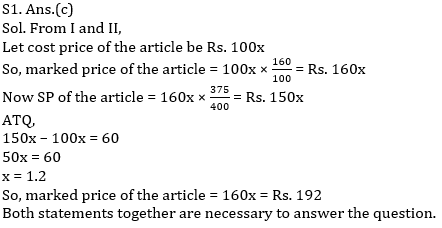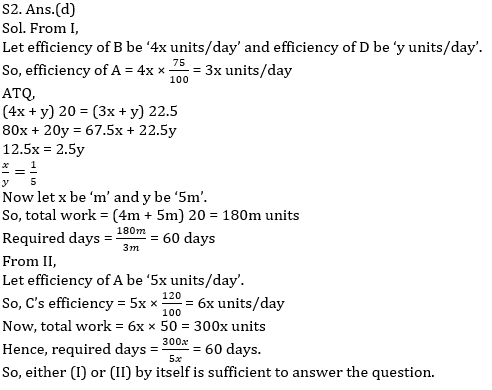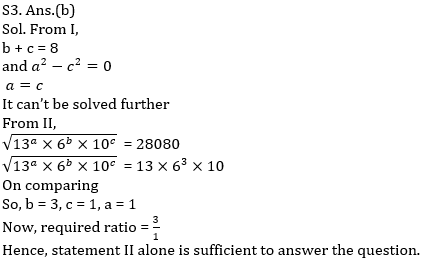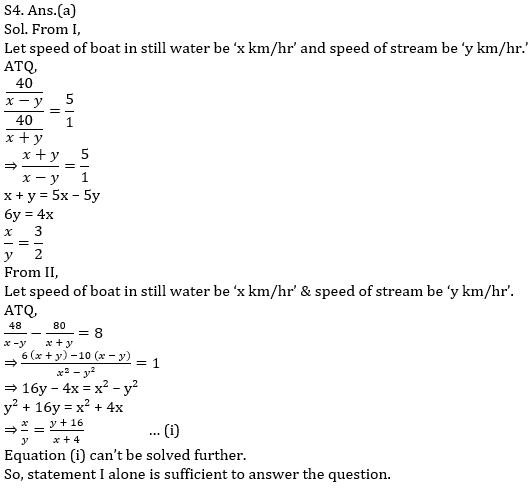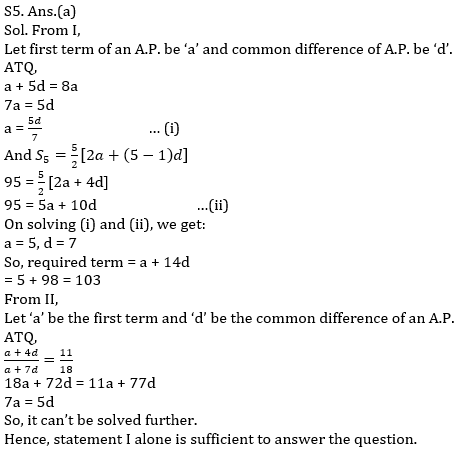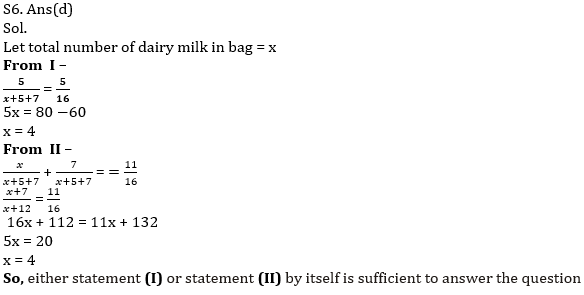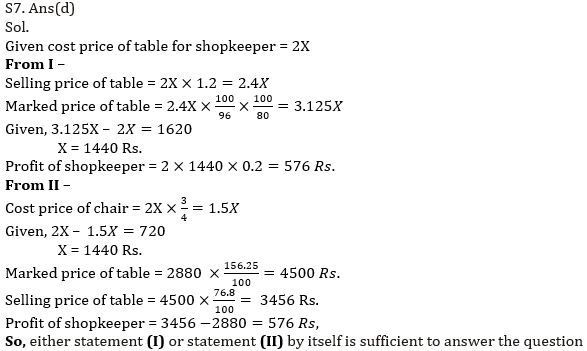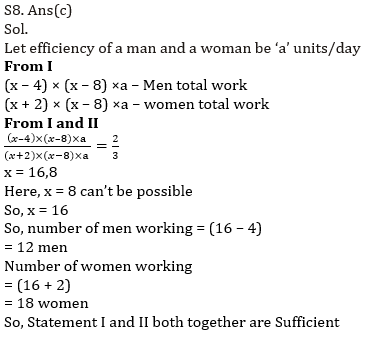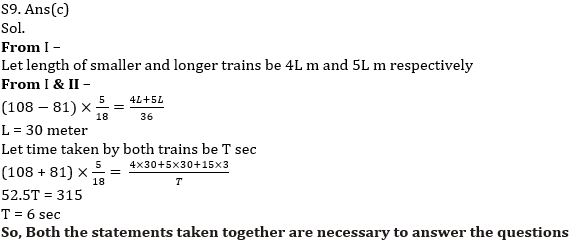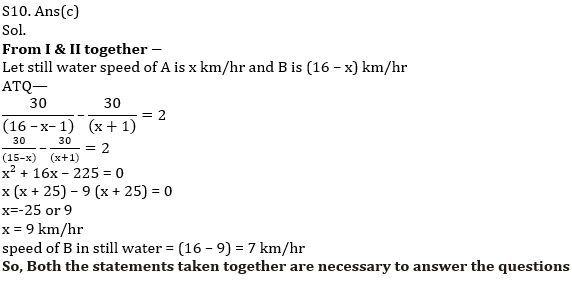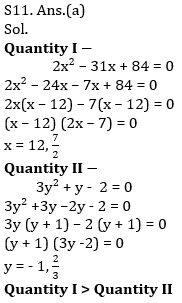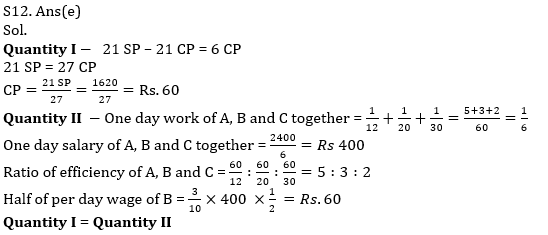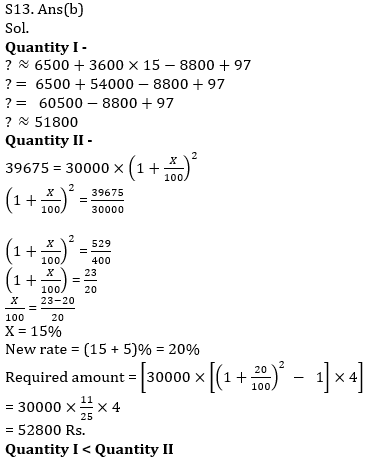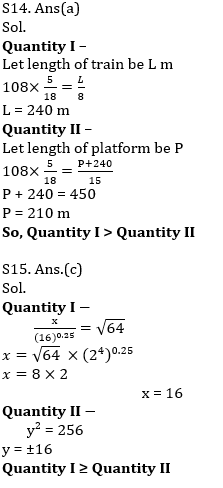×

Thank You, Your details have been submitted we will get back to you.Join India's largest learning destination

What You Will get ?

•Job Alerts
•Daily Quizzes
•Subject-Wise Quizzes
•Current Affairs
•Previous year question papers
•Doubt Solving session

ORJoin India's largest learning destination

What You Will get ?

•Job Alerts
•Daily Quizzes
•Subject-Wise Quizzes
•Current Affairs
•Previous year question papers
•Doubt Solving session

ORJoin India's largest learning destination

What You Will get ?

•Job Alerts
•Daily Quizzes
•Subject-Wise Quizzes
•Current Affairs
•Previous year question papers
•Doubt Solving session

Enter the email address associated with your account, and we'll email you an OTP to verify it's you.Join India's largest learning destination

What You Will get ?

•Job Alerts
•Daily Quizzes
•Subject-Wise Quizzes
•Current Affairs
•Previous year question papers
•Doubt Solving session

Enter OTP

Please enter the OTP sent to
/6

Did not recive OTP?

Resend in 60sJoin India's largest learning destination

What You Will get ?

•Job Alerts
•Daily Quizzes
•Subject-Wise Quizzes
•Current Affairs
•Previous year question papers
•Doubt Solving sessionJoin India's largest learning destination

What You Will get ?

•Job Alerts
•Daily Quizzes
•Subject-Wise Quizzes
•Current Affairs
•Previous year question papers
•Doubt Solving session

Almost there

+91Join India's largest learning destination

What You Will get ?

•Job Alerts
•Daily Quizzes
•Subject-Wise Quizzes
•Current Affairs
•Previous year question papers
•Doubt Solving session

Enter OTP

Please enter the OTP sent to Edit Number

Did not recive OTP?

Resend 60

By skipping this step you will not recieve any free content avalaible on adda247, also you will miss onto notification and job alerts

Are you sure you want to skip this step?

By skipping this step you will not recieve any free content avalaible on adda247, also you will miss onto notification and job alerts

Are you sure you want to skip this step?